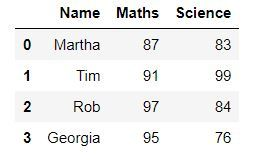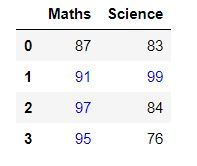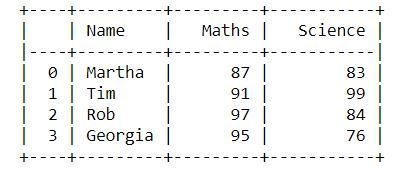Open In App

# Display the Pandas DataFrame in table style

In this article, we’ll see how we can display a DataFrame in the form of a table with borders around rows and columns. It’s necessary to display the DataFrame in the form of a table as it helps in proper and easy visualization of the data. Now, let’s look at a few ways with the help of examples in which we can achieve this.

Example 1: One way to display a dataframe in the form of a table is by using the `display()` function of `IPython.display`.

## Python3

 `# importing the modules``from` `IPython.display ``import` `display``import` `pandas as pd` `# creating a DataFrame``dict` `=` `{``'Name'` `: [``'Martha'``, ``'Tim'``, ``'Rob'``, ``'Georgia'``],``        ``'Maths'` `: [``87``, ``91``, ``97``, ``95``],``        ``'Science'` `: [``83``, ``99``, ``84``, ``76``]}``df ``=` `pd.DataFrame(``dict``)` `# displaying the DataFrame``display(df)`

Output :Example 2: In this example we’ll use `DataFrame.style`. It returns a Styler object, which has useful methods for formatting and displaying DataFrames.

## Python3

 `# importing the module``import` `pandas as pd` `# creating a DataFrame``dict` `=` `{``'Name'` `: [``'Martha'``, ``'Tim'``, ``'Rob'``, ``'Georgia'``],``        ``'Maths'` `: [``87``, ``91``, ``97``, ``95``],``        ``'Science'` `: [``83``, ``99``, ``84``, ``76``]}``df ``=` `pd.DataFrame(``dict``)` `# displaying the DataFrame``df.style`

Output :Example 3 : Using `DataFrame.style` we can also add different styles to our dataframe table. Like, in this example we’ll display all the values greater than 90 using the blue colour and rest with black. To achieve this we’ll use `DataFrame.style.applymap()` to traverse through all the values of the table and apply the style.

## Python3

 `# importing the modules``import` `pandas as pd``import` `numpy as np` `def` `color_negative_red(val):``    ``"""``    ``Takes a scalar and returns a string with``    ``the css property `'color: red'` for negative``    ``strings, black otherwise.``    ``"""``    ``color ``=` `'blue'` `if` `val > ``90` `else` `'black'``    ``return` `'color: % s'` `%` `color` `# creating a DataFrame``dict` `=` `{``'Maths'` `: [``87``, ``91``, ``97``, ``95``],``        ``'Science'` `: [``83``, ``99``, ``84``, ``76``]}``df ``=` `pd.DataFrame(``dict``)` `# displaying the DataFrame``df.style.applymap(color_negative_red)`

Output :Example 4 : We can also use a library called tabulate for this purpose. It is a library which contains different styles in which dataframes can be displayed. In this example we’ll use the `"psql"` style.

## Python3

 `# importing the modules``from` `tabulate ``import` `tabulate``import` `pandas as pd` `# creating a DataFrame``dict` `=` `{``'Name'``:[``'Martha'``, ``'Tim'``, ``'Rob'``, ``'Georgia'``],``        ``'Maths'``:[``87``, ``91``, ``97``, ``95``],``        ``'Science'``:[``83``, ``99``, ``84``, ``76``]}``df ``=` `pd.DataFrame(``dict``)` `# displaying the DataFrame``print``(tabulate(df, headers ``=` `'keys'``, tablefmt ``=` `'psql'``))`

Output :Below are all the styles that you can use :

• “plain”
• “simple”
• “github”
• “grid”
• “fancy_grid”
• “pipe”
• “orgtbl”
• “jira”
• “presto”
• “pretty”
• “psql”
• “rst”
• “mediawiki”
• “moinmoin”
• “youtrack”
• “html”
• “latex”
• “latex_raw”
• “latex_booktabs”
• “textile”